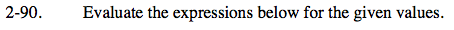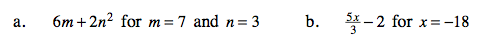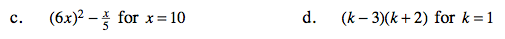### Home > CAAC > Chapter 2 > Lesson 2.1.9 > Problem2-90

2-90.Substitute the given values for the variables m and n.

6(7) + 2(32)

Simplify the expression.
42 + 18 = 60

60

Substitute −18 for x in the expression. Then simplify.After substituting 10 for x, the expression becomes:

$(6\cdot10)^{2}-\frac{10}{5}$

Simplify. Be sure to multiply the 6 and 10 before squaring!

Replace each k with 1, and then simplify the expression.

−6